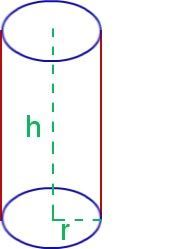# Cylinder Formula

## CYLINDER FORMULA

Cylinder is a stable structure comprising of two parallel faces and two circular bases. The radius of the circular base is the distance between the center of the base to the edge of the cylinder. The two bases are perpendicular to the two faces of the cylinder.

The Cylinder Formula is applied to calculate the surface area and volume of the cylinder.The Cylinder Formula is expressed as,

Total Surface area of a cylinder = 2πr(r + h)

Curved Surface Area = 2πrh

Volume of a cylinder = πr2h

Where in,

r denotes the radius of the circular base of the cylinder.

h indicates the height of the parallel face of the cylinder.

Example 1:

A cylinder has base radius 5 cm and height 7 cm. Calculate the total surface area and volume of the cylinder.

Solution:

Given parameters are,

r is 5 cm

h is 7 cm

Surface area of a cylinder is given by,

= 2πr(r + h)

= 2π x 5 (5 + 7)

= 2π x 5 x 12

= 377.04 cm2

Volume of a cylinder is given by

πr2h

= π (5)2 x 7

= π x 25 x 7

= 549.85 cm3

Example 2:

The base radius of a cylinder is 11 cm, and the height is 20 cm. Calculate the total surface area and volume of the cylinder.

Solution:

Given parameters are,

r is 11 cm

h is 20 cm

Surface area of the cylinder is expressed by

2πr(r + h)

= 2π x11(11 + 20)

= 2π x 11 x 31

= 2142.844 cm2

Volume of the cylinder is given by

πr2h

= π(11)2 x 20

= π x 121 x 20

= 7603.64 cm3